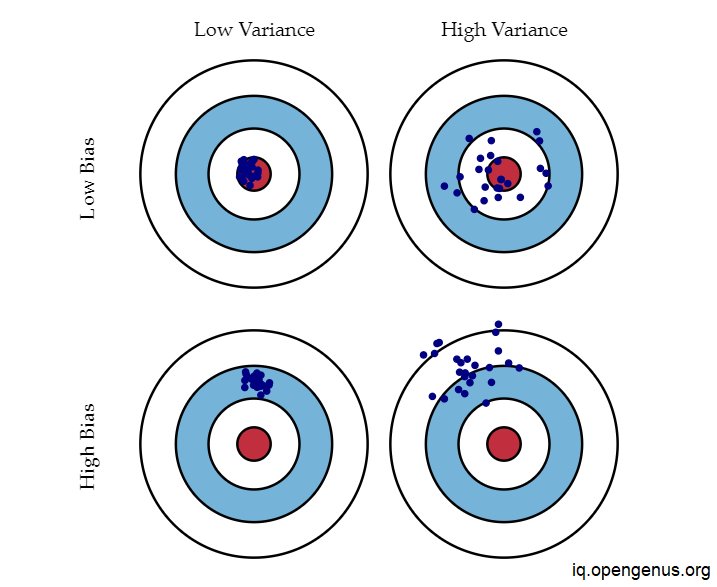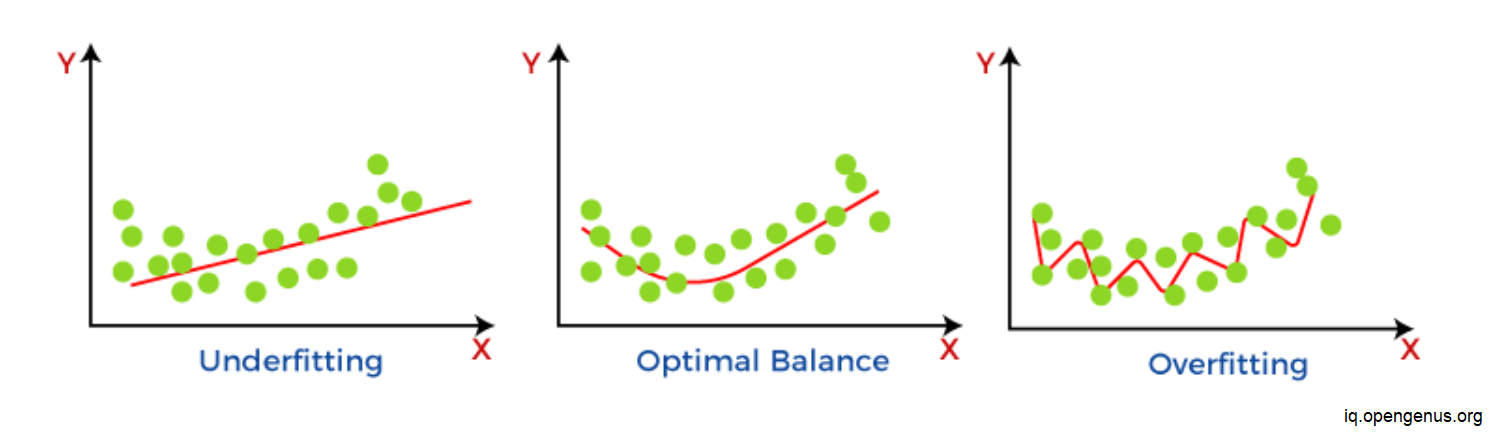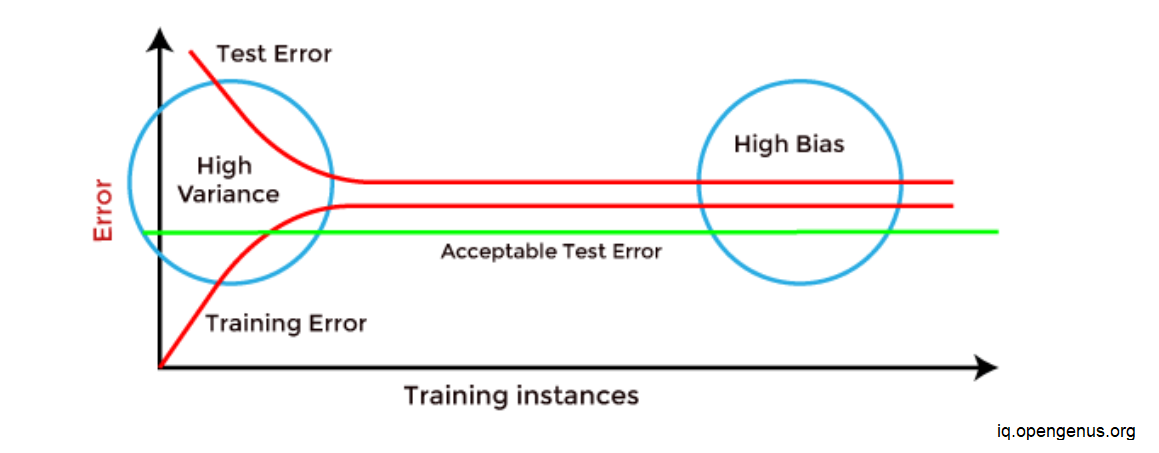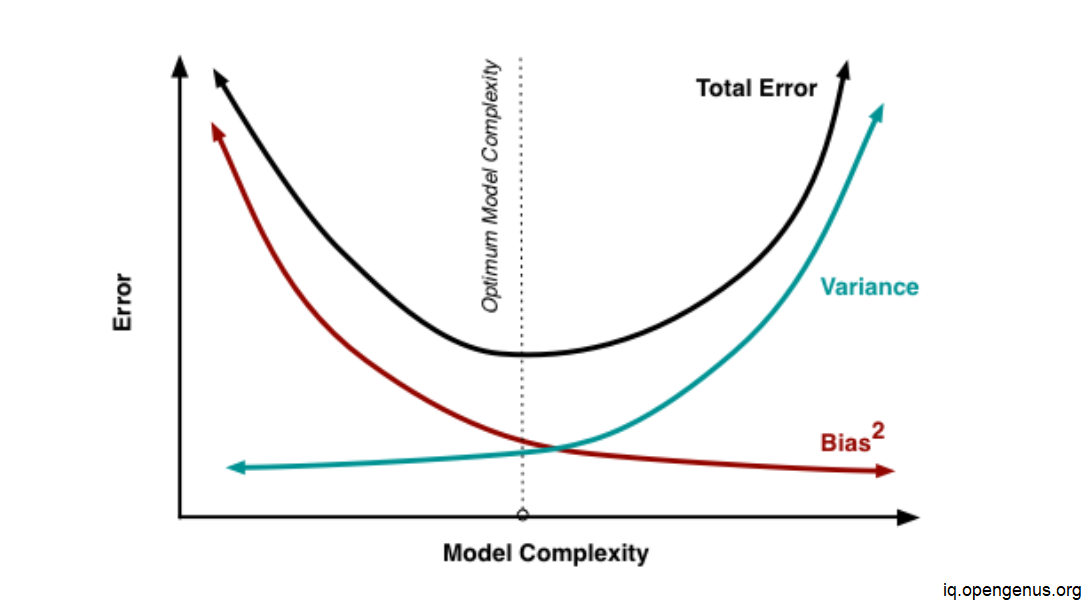×

Search anything:

#### Machine Learning (ML) Deep LearningAn essential idea in statistical learning and machine learning is the bias-variance tradeoff. It speaks to the connection between a model's complexity and its precision in fitting the data. It specifically discusses the compromise between a model's bias, or systematic error, and variance, or random error.

## Bias

Bias is the discrepancy between the target variable's actual value and the expected value of a model's predictions. Because it does not accurately reflect the real underlying relationship between the characteristics and the target, a model with significant bias tends to underfit the data. To put it another way, the model is too basic and can't adequately represent the complexity of the data.

## Variance

Variance is the variability of a model's forecast for a certain data point or value, which indicates how widely distributed our data are. A model with a large variance pays close attention to the training data and does not generalize to new data. As a result, these models have significant error rates on test data yet perform quite well on training data.

Mathematically

Let Y stand for the variable we are attempting to forecast and X stand for other covariates. Since we presume that there is a connection between the two,

Y = f(x) + e

Where e is the error term and it’s normally distributed with a mean of 0.
We will make a model f^(X) of f(X) using linear regression or any other modeling technique.

So the expected squared error at a point x is

Err(x) = Bias^2 + Variance + Irreducible error

Err(x) is the sum of Bias², variance and the irreducible error.

Bias and variance using bulls-eye diagramA model that accurately predicts the right values is the goal in the diagram above. Our forecasts get progressively worse as we get further from the bulls-eye. We can repeat the model-building process to get additional hits on the target.
When a model in supervised learning is unable to catch the underlying pattern of the data, underfitting occurs. These models typically have minimal variance and high bias. It occurs when we attempt to create a linear model using nonlinear data or when we have very little data on which to base an accurate model. Additionally, these kinds of models, like logistic and linear regression, are very straightforward to represent the complex patterns in data.
Overfitting occurs in supervised learning when our model includes both the background noise and the underlying pattern. This occurs when we teach our model repeatedly on noisy data. These models have significant variance and little bias. These models are extremely complicated and prone to overfitting, like decision trees.1. Low-Bias, Low-Variance:
The combination of low bias and low variance shows an ideal machine learning model. However, it is not possible practically.
2. Low-Bias, High-Variance:
With low bias and high variance, model predictions are inconsistent and accurate on average. This case occurs when the model learns with a large number of parameters and hence leads to an overfitting
3. High-Bias, Low-Variance:
With High bias and low variance, predictions are consistent but inaccurate on average. This case occurs when a model does not learn well with the training dataset or uses few numbers of the parameter. It leads to underfitting problems in the model.
4. High-Bias, High-Variance:
With high bias and high variance, predictions are inconsistent and also inaccurate on average.

How to identify High variance or High Bias?High variance can be identified if the model has:
Low training error and high test error.

High Bias can be identified if the model has:
High training error and the test error is almost similar to training error.

In order to prevent overfitting and underfitting in the machine learning model, bias and variation must be carefully considered while the model is being built. A very basic model with few parameters may have a high bias and low variance. In contrast, a model with many factors will have a high variance and a low bias. Therefore, it is necessary to strike an equilibrium between the bias error and variance error; this balance is known as the bias-variance trade-off.A low variance and low bias are required by algorithms for a model's prediction to be correct. However, due to the relationship between prejudice and variance, this is not possible:

• If we decrease the variance, it will increase the bias.
• If we decrease the bias, it will increase the variance.

In supervised learning, the trade-off between bias and variance is crucial. The ideal model would correctly represent the regularities in the training set while also generalizing well to the unknown dataset. Unfortunately, this cannot be done at the same time. Due to the possibility of overfitting to noisy data, a high variance algorithm may work well with training data. In contrast, a high bias algorithm creates a much simpler model that might even miss crucial data regularities. Therefore, in order to create the best model, we must discover the ideal balance between bias and variance.

As a result, the Bias-Variance trade-off involves locating the optimal equilibrium between bias and variance mistakes.